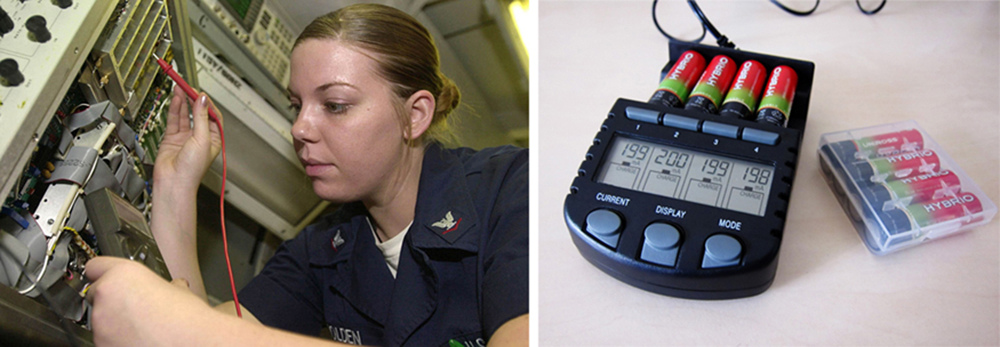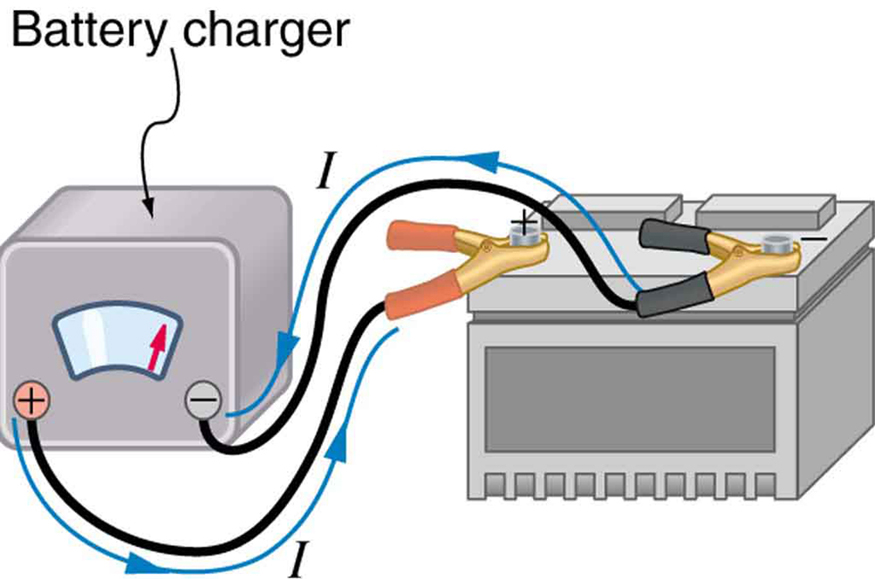# 21.2 Electromotive force: terminal voltage  (Page 4/12)

 Page 4 / 12
$\begin{array}{lll}V& =& \text{emf}-\text{Ir}=\text{12.0 V}-\left(\text{1.188 A}\right)\left(\text{0.100 Ω}\right)\\ & =& \text{11.9 V.}\end{array}$

Discussion for (a)

The terminal voltage here is only slightly lower than the emf, implying that $\text{10}\text{.}0\phantom{\rule{0.25em}{0ex}}\Omega$ is a light load for this particular battery.

Solution for (b)

Similarly, with ${R}_{\text{load}}=0\text{.}\text{500}\phantom{\rule{0.15em}{0ex}}\Omega$ , the current is

$I=\frac{\text{emf}}{{R}_{\text{load}}+r}=\frac{\text{12}\text{.}0\phantom{\rule{0.25em}{0ex}}\text{V}}{0\text{.}\text{600}\phantom{\rule{0.15em}{0ex}}\Omega }=\text{20}\text{.}0\phantom{\rule{0.25em}{0ex}}\text{A}.$

The terminal voltage is now

$\begin{array}{lll}V& =& \text{emf}-\text{Ir}=\text{12.0 V}-\left(\text{20.0 A}\right)\left(\text{0.100 Ω}\right)\\ & =& \text{10}\text{.}\text{0 V.}\end{array}$

Discussion for (b)

This terminal voltage exhibits a more significant reduction compared with emf, implying $0\text{.}\text{500}\phantom{\rule{0.15em}{0ex}}\Omega$ is a heavy load for this battery.

Solution for (c)

The power dissipated by the $0\text{.}\text{500 - Ω}$ load can be found using the formula $P={I}^{2}R$ . Entering the known values gives

${P}_{\text{load}}={I}^{2}{R}_{\text{load}}={\left(\text{20.0}\phantom{\rule{0.25em}{0ex}}\text{A}\right)}^{2}\left(0\text{.500 Ω}\right)=2.00×{10}^{2}\phantom{\rule{0.25em}{0ex}}\text{W}.$

Discussion for (c)

Note that this power can also be obtained using the expressions $\frac{{V}^{2}}{R}$ or $\text{IV}$ , where $V$ is the terminal voltage (10.0 V in this case).

Solution for (d)

Here the internal resistance has increased, perhaps due to the depletion of the battery, to the point where it is as great as the load resistance. As before, we first find the current by entering the known values into the expression, yielding

$I=\frac{\text{emf}}{{R}_{\text{load}}+r}=\frac{\text{12}\text{.}0\phantom{\rule{0.25em}{0ex}}\text{V}}{1\text{.}\text{00}\phantom{\rule{0.25em}{0ex}}\Omega }=\text{12}\text{.}0\phantom{\rule{0.25em}{0ex}}\text{A}.$

Now the terminal voltage is

$\begin{array}{lll}V& =& \text{emf}-\text{Ir}=\text{12.0 V}-\left(\text{12.0 A}\right)\left(\text{0.500 Ω}\right)\\ & =& \text{6.00 V,}\end{array}$

and the power dissipated by the load is

${P}_{\text{load}}={I}^{2}{R}_{\text{load}}={\left(\text{12.0 A}\right)}^{2}\left(0\text{.}\text{500}\phantom{\rule{0.25em}{0ex}}\Omega \right)=\text{72}\text{.}0\phantom{\rule{0.25em}{0ex}}\text{W}.$

Discussion for (d)

We see that the increased internal resistance has significantly decreased terminal voltage, current, and power delivered to a load.

Battery testers, such as those in [link] , use small load resistors to intentionally draw current to determine whether the terminal voltage drops below an acceptable level. They really test the internal resistance of the battery. If internal resistance is high, the battery is weak, as evidenced by its low terminal voltage.These two battery testers measure terminal voltage under a load to determine the condition of a battery. The large device is being used by a U.S. Navy electronics technician to test large batteries aboard the aircraft carrier USS Nimitz and has a small resistance that can dissipate large amounts of power. (credit: U.S. Navy photo by Photographer’s Mate Airman Jason A. Johnston) The small device is used on small batteries and has a digital display to indicate the acceptability of their terminal voltage. (credit: Keith Williamson)

Some batteries can be recharged by passing a current through them in the direction opposite to the current they supply to a resistance. This is done routinely in cars and batteries for small electrical appliances and electronic devices, and is represented pictorially in [link] . The voltage output of the battery charger must be greater than the emf of the battery to reverse current through it. This will cause the terminal voltage of the battery to be greater than the emf, since $V=\text{emf}-\text{Ir}$ , and $I$ is now negative.A car battery charger reverses the normal direction of current through a battery, reversing its chemical reaction and replenishing its chemical potential.

## Multiple voltage sources

There are two voltage sources when a battery charger is used. Voltage sources connected in series are relatively simple. When voltage sources are in series, their internal resistances add and their emfs add algebraically. (See [link] .) Series connections of voltage sources are common—for example, in flashlights, toys, and other appliances. Usually, the cells are in series in order to produce a larger total emf.

full meaning of GPS system
how to prove that Newton's law of universal gravitation F = GmM ______ R²
sir dose it apply to the human system
prove that the centrimental force Fc= M1V² _________ r
prove that centripetal force Fc = MV² ______ r
Kaka
how lesers can transmit information
griffts bridge derivative
below me
please explain; when a glass rod is rubbed with silk, it becomes positive and the silk becomes negative- yet both attracts dust. does dust have third types of charge that is attracted to both positive and negative
what is a conductor
Timothy
hello
Timothy
below me
why below you
Timothy
no....I said below me ...... nothing below .....ok?
dust particles contains both positive and negative charge particles
Mbutene
corona charge can verify
Stephen
when pressure increases the temperature remain what?
remains the temperature
betuel
what is frequency
define precision briefly
CT scanners do not detect details smaller than about 0.5 mm. Is this limitation due to the wavelength of x rays? Explain.
hope this helps
what's critical angle
The Critical Angle Derivation So the critical angle is defined as the angle of incidence that provides an angle of refraction of 90-degrees. Make particular note that the critical angle is an angle of incidence value. For the water-air boundary, the critical angle is 48.6-degrees.
okay whatever
Chidalu
pls who can give the definition of relative density?
Temiloluwa
the ratio of the density of a substance to the density of a standard, usually water for a liquid or solid, and air for a gas.
Chidalu
What is momentum
mass ×velocity
Chidalu
it is the product of mass ×velocity of an object
Chidalu
how do I highlight a sentence]p? I select the sentence but get options like copy or web search but no highlight. tks. src
then you can edit your work anyway you want
Wat is the relationship between Instataneous velocity
Instantaneous velocity is defined as the rate of change of position for a time interval which is almost equal to zero
AstronomyByByBy John GabrieliBy Lakeima RobertsBy OpenStaxBy Brenna FikeBy OpenStaxBy Mahee BooBy Mary MateraBy Yasser IbrahimBy OpenStaxBy Janet Forrester